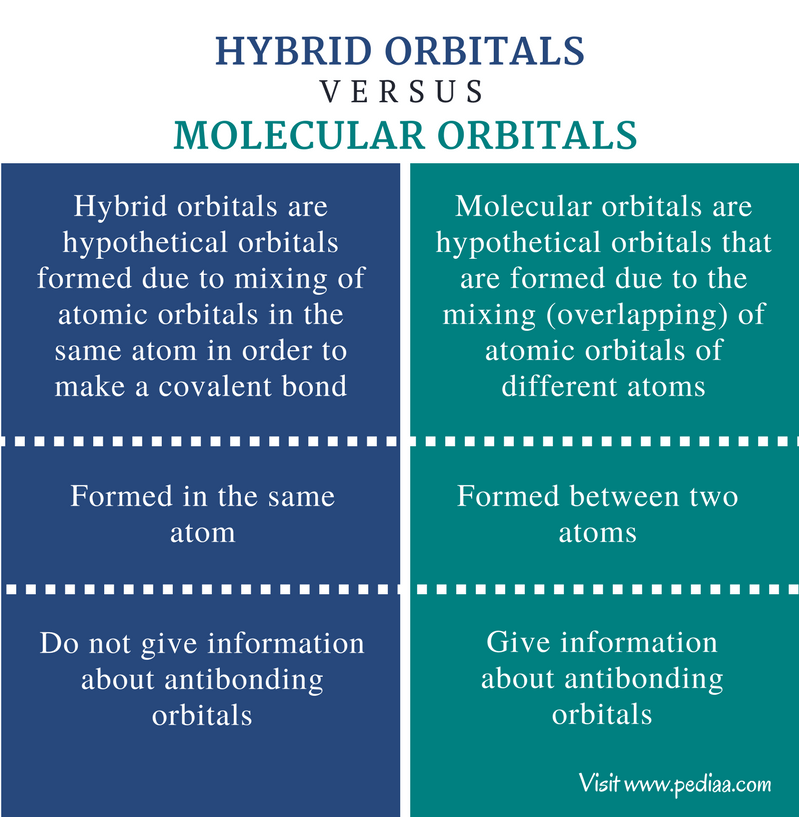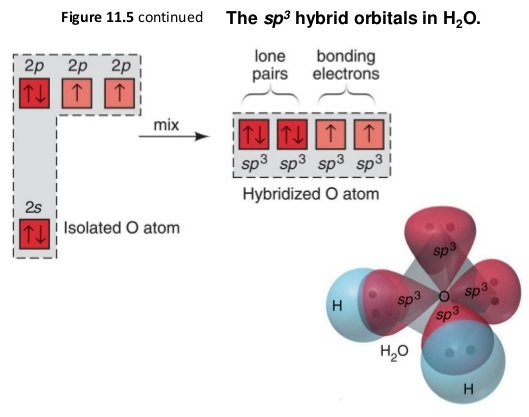# Difference Between Hybrid Orbitals and Molecular Orbitals

## Main Difference – Hybrid Orbitals vs Molecular Orbitals

Orbitals are hypothetical structures that can be filled with electrons. According to different discoveries, scientists have proposed different shapes for these orbitals. There are three main types of orbitals: atomic orbitals, molecular orbitals, and hybrid orbitals. Atomic orbitals are the hypothetical orbitals that are located around the nucleus of an atom. Molecular orbitals are the hypothetical orbitals formed when two atoms make a covalent bond between them. Hybrid orbitals are hypothetical orbitals that are formed due to the hybridization of atomic orbitals. The main difference between hybrid orbitals and molecular orbitals is that hybrid orbitals are formed by the interactions of atomic orbitals in the same atom while molecular orbitals are formed by the interactions of atomic orbitals of two different atoms.

### Key Areas Covered

1. What are Hybrid Orbitals
– Formation, Shapes and Properties
2. What are Molecular Orbitals
– Formation, Shapes and Properties
3. What are the Similarities Between Hybrid Orbitals and Molecular Orbitals
– Outline of Common Features
4. What is the Difference Between Hybrid Orbitals and Molecular Orbitals
– Comparison of Key Differences

Key Terms: Antibonding Molecular Orbital, Atomic Orbital, Bonding Molecular Orbital, Hybridization, Hybrid Orbital, Molecular Orbital## What are Hybrid Orbitals

Hybrid orbitals are hypothetical orbitals formed due to mixing of atomic orbitals in the same atom in order to make a covalent bond. In other words, atomic orbitals of an atom undergo hybridization to make suitable orbitals for chemical bonding. Atomic orbitals are found as s orbital, p orbital, d orbital and f orbital. Hybridization of two or more orbitals will form a new hybrid orbital. Hybrid orbitals are named according to the atomic orbitals that undergo hybridization. Some examples are given below.

### sp Hybrid Orbital

These orbitals are formed when one s orbital and one p orbital are mixed. The resulting hybrid orbitals have 50% of s characteristics and 50% of p characteristics. The spatial arrangement of sp orbitals is linear. Therefore, the bond angle between these orbitals is 180oC. The atoms that undergo sp hybridization have 2 empty p orbitals.

### sp2 Hybrid Orbital

These orbitals are formed when one s orbital and 2 p orbitals are hybridized. The resulting hybrid orbitals have about 33% of s characters and about 66% of p characters. The spatial arrangement of these orbitals is trigonal planar. Therefore, the bond angle between these orbitals is 120oC. The atoms that undergo this hybridization have 1 empty p orbital.

### sp3 Orbital

These orbitals are formed when one s orbital and 3 p orbitals are hybridized. The resulting hybrid orbitals have about 25% of s characters and about 75% of p characters. The spatial arrangement of these orbitals is tetrahedral. Therefore, the bond angle between these orbitals is 109.5oC. The atoms that undergo this hybridization have no empty p orbitals.

### sp3d1 Orbital

These orbitals are formed when one s orbital, 3 p orbitals and one d orbital are hybridized. The spatial arrangement of these orbitals is trigonal planar. The atoms that undergo this hybridization have 4 empty d orbitals.Figure 1: sp3 hybridization of H2O molecule

The above image shows the hybridization of atomic orbitals of the oxygen molecule in order to form two covalent bonds with two hydrogen atoms.

## What are Molecular Orbitals

Molecular orbitals are hypothetical orbitals that are formed due to the mixing (overlapping) of atomic orbitals of different atoms. This occurs when a covalent bond is formed between two atoms. For example, if a covalent bond is formed between A and B atoms, the atomic orbitals that have the correct symmetry will be mixed, forming a molecular orbital. Therefore, molecular orbitals are the regions where most of the bonding electrons can be found between two atoms. Molecular orbitals can be found in two types as bonding orbitals and antibonding orbitals.

### Bonding Molecular Orbitals

These orbitals have less energy when compared to atomic orbitals that undergo formation of the molecular orbital. Therefore, these orbitals are stable. The bond electron pair can be found in this orbital.

### Antibonding Molecular Orbitals

These orbitals have a higher energy than atomic orbitals and bonding molecular orbitals. Therefore they are less stable. Most of the times, these orbitals are empty.Figure 2: The Molecular Orbital Diagram of O2 Molecule

The above image shows the molecular orbital diagram for diatomic oxygen. The symbol “σ” indicates sigma bonding molecular orbital and “σ*” indicates the antibonding orbital.

## Similarities Between Hybrid Orbitals and Molecular Orbitals

• Hybrid orbitals and molecular orbitals are formed due to the mixing of atomic orbitals.
• Both types of orbitals show the most probable location of the bond electron pair.

## Difference Between Hybrid Orbitals and Molecular Orbitals

### Definition

Hybrid Orbitals: Hybrid orbitals are hypothetical orbitals formed due to mixing of atomic orbitals in the same atom in order to make a covalent bond.

Molecular Orbitals: Molecular orbitals are hypothetical orbitals that are formed due to the mixing (overlapping) of atomic orbitals of different atoms.

### Atoms

Hybrid Orbitals:  Hybrid orbitals are formed in the same atom.

Molecular Orbitals:  Molecular orbitals are formed between two atoms.

### Antibonding Orbital

Hybrid Orbitals:  Hybrid orbitals do not give information about antibonding orbitals.

Molecular Orbitals: Molecular orbitals give information about antibonding orbitals.

### Conclusion

Both hybrid orbitals and molecular orbitals are hypothetical orbitals that show the most probable location of electrons in atoms or in between atoms. They are very important in predicting the shape of a molecule. The main difference between hybrid orbitals and molecular orbitals is that hybrid orbitals are formed by the interactions of atomic orbitals in the same atom while molecular orbitals are formed by the interactions of atomic orbitals of two different atoms.

##### References:

1. Libretexts. “Hybrid Orbitals.” Chemistry LibreTexts. Libretexts, 21 July 2016. Web. Available here. 14 Aug. 2017.
2. Libretexts. “How to Build Molecular Orbitals.” Chemistry LibreTexts. Libretexts, 21 July 2016. Web. Available here. 14 Aug. 2017.

##### Image Courtesy:

1. “Sp3 hybridization of H2O” By Holmescallas – Own work (CC BY-SA 4.0) via Commons Wikimedia
2. “Oxygen molecule orbitals diagram” By Anthony. Sebastian –  (CC BY-SA 3.0) via Commons Wikimedia